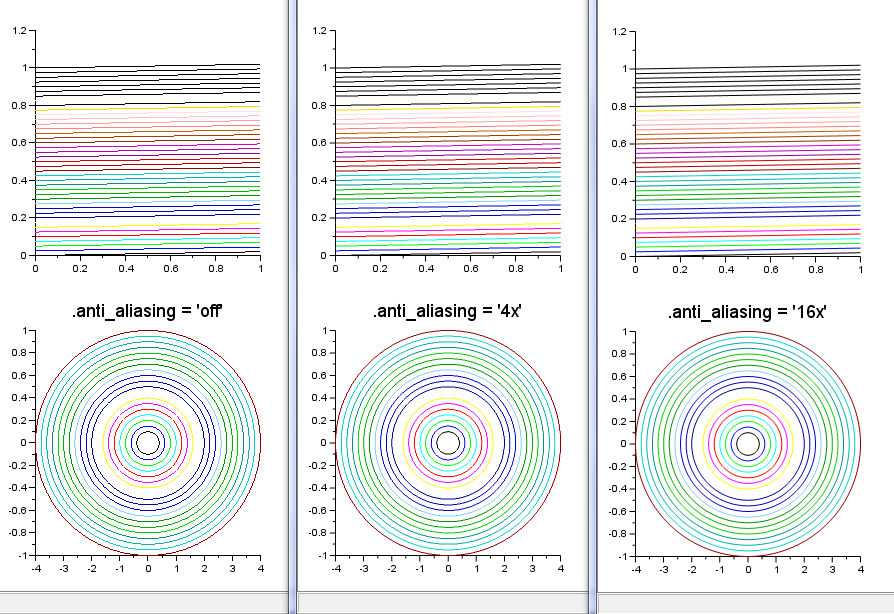Scilab Home page | Wiki | Bug tracker | Forge | Mailing list archives | ATOMS | File exchange
Change language to: English - Français - 日本語 - Русский
Ajuda do Scilab >> Biblioteca de Gráficos > figure_operations > anti_aliasing

# anti_aliasing

Figure's property smoothing the stair-like aspect when rendering curves

### Description

When some curves or parts of curves have a small slope with respect to the horizontal or vertical direction of ranges of screen pixels, their raw rendering shows a stair-like aspect.

Figures have an `.anti_aliasing` property that can be used to smooth this rendering effect. Possible values are `'off'`, `'2x'`, `'4x'`, `'8x'`, or `'16x'`.Enabling the anti-aliasing decreases the rendering speed of graphics. In average, the time spent to build and display graphics varies approximately according to the following factors:
 .anti_aliasing time spent off 2x 4x 8x 16x 1 1.4 2.1 3.6 6.6

### Examples

```x = [0 1];
y = (0:0.025:1)';
y = [y y+0.02]';

th = 0:2:360;
xe = 4*cosd(th); xe = (0.1:0.05:1)' * xe;
ye = sind(th);   ye = (0.1:0.05:1)' * ye;

usecanvas(%t);

i = 0;
for aa = ['off' '4x' '16x']
f = scf(i);
f.axes_size = [300 600];
f.figure_position(1) = 100+300*i;
f.anti_aliasing = aa;  // <<<<<<<<
clf
subplot(2,1,1)
plot2d(x,y)
subplot(2,1,2)
plot2d(xe',ye')
title(".anti_aliasing = ''"+aa+"''", "fontsize",4);
i = i+1;
end```